# Mixtures and solutions + volume - math problems

1. Water mixingThere are 5 liters of water at 75°C in the pot, how much water at 10°C do we have to add to the resulting temperature of 55°C?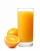Iva added one liter of 100% fruit juice to 3 liters of water. She left two liters of it for Alice and Beata. She added two more liters of water to the remain two liters of lemonade and offered it to other friends. a. What percentage of juice did Alena an
3. Aircraft angines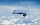The two engines of the aircraft are enough to supply the fuel for five hours of operation. However, one of the engines has a malfunction and thus consumes one-third more fuel. How long can the plane be in the air before it runs out of fuel? After an hour
4. Pouring alcohol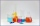100 liters of alcohol has 70% How many liters of water need to be added to have 60% alcohol?
5. Alcohol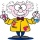6l 48% alcohol, how much 52% alcohol must be added to give 50% alcohol?
6. Clogging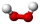How much distilled water must the pharmacist add to 30g of a 30% hydrogen peroxide solution to obtain a 3% solution to clogging?
7. The pool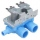The pool contains 220 m3 of water. The pool can be emptied either: a) 10 hours of pipe B and 8 hours of pipe A, or b) 10 hours of pipe A and 7 hours of pipe B. How many cubic meters of water will flow in 1 hour from pipe A and how many from pipe B?
8. Mixing waterThe 30-liter container should we fill with water at 60 degrees Celsius. How many liters of water 80 degrees C hot and how many liters of water 20 degrees Celsius warm we have to mix?
9. Home cleaning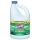Mr. Smith is cleaning up a big mess at home. In the closet, he finds a solution that is 5% bleach, and another stronger solution that is 20% bleach. For this particular job, he needs 600mL of 15% bleach. How much of each type (to the nearest mL) should he
10. PeroxideHow many ml 30% of peroxide (H2O2) should be poured into 100ml H2O to give a 20% solution?
11. Solutions, mixturesHow many liters of 70% solution we must add to 5 liters of 30% solution to give us a 60% solution?
12. Fruit juice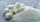Fruit juice contains 37.5% sugar. How many percents of sugar will be in the flavored mineral that we prepare from 100 grams of fruit juice and 1.4 liters of mineral? (1 liter = 1 kg)
13. Mixture 2How many liters of water must be added to 7 liters of a 20% solution to obtain a 10% solution?
14. MixtureHow many percentages mixtures do we get by adding 23ml of matter to 89ml of water?
15. Alcohol mixtureThree liters of 96 percent alcohol we set up a certain amount of distilled water to form a 54-percent alcohol. How many liters of distilled water were used?
16. The poolThe pool has a volume of 40 m3 and the water temperature is 20 °C. How much water at 100 °C should we pour into the pool to increase the water temperature by 5 °C?
17. CoolantThe driver of the car cooler filled with a mixture of 3.9 liters and 2.6 liters of water antifreeze coolant. At what rate are this two components of the mixture?
18. PharmacyThe pharmacy add to 3 liters of 95-percent alcohol 5 liters of 38.04 percent alcohol. How many percent pharmacy got?
19. CuZn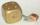Brass is an alloy of copper and zinc. The 10 centimeter brass cube has a weight of 8.6 kg. Copper density is 8930 kg/m3, the zinc density is 7130 kg/m3. Calculate how many kg of copper and zinc a cube contains.
20. Hydrochloric acidDetermine the concentration of which must have a solution of hydrochloric acid that mixing 10 l of the solution with 8 liters of 26% solution to get the solution with a concentration of 50%?

Do you have an interesting mathematical word problem that you can't solve it? Submit math problem, and we can try to solve it.

We will send a solution to your e-mail address. Solved examples are also published here. Please enter the e-mail correctly and check whether you don't have a full mailbox.

Please do not submit problems from current active competitions such as Mathematical Olympiad, correspondence seminars etc...

Tip: Our volume units converter will help you with the conversion of volume units.